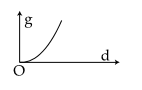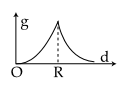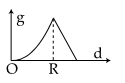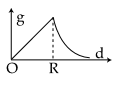#### The variation of acceleration due to gravity g with distance d from centre of the earth is best represented by(R=Earth’s radius) : Option 1)Option 2)Option 3)Option 4)As we learnt in

Value of 'g' at ∞ -ifValue of acceleration due to gravityheight above earth's surface

- wherein

No effect of earth gravitational pull at infinite distance.

ifi.e.Option 1)Incorrect

Option 2)Incorrect

Option 3)Incorrect

Option 4)Correct

#### Sabhrant Ambastha